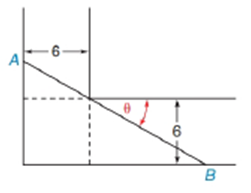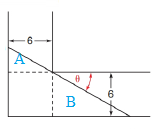Chapter 11.2, Problem 40EElementary Geometry For College St...

7th Edition
Alexander + 2 others
ISBN: 9781337614085

Solutions

Chapter
SectionElementary Geometry For College St...

7th Edition
Alexander + 2 others
ISBN: 9781337614085
Textbook Problem

A ladder of length AB is carried horizontally through an L-shaped turn in a hallway. Show that the ladder has the length L = 6 sin θ + 6 cos θTo determine

To find:

To show that the ladder has the length L=6sinθ+6cosθ by using the following figure,Explanation

Consider the following figure,

General formula for cosine ratio and sin ratio is given below,

For smaller triangle A:

From the above figure shows that the hypotenuse A of the smaller triangle is formed by the left end of the ladder since it is opposite to right angle

Still sussing out bartleby?

Check out a sample textbook solution.

See a sample solution

The Solution to Your Study Problems

Bartleby provides explanations to thousands of textbook problems written by our experts, many with advanced degrees!

Get Started

Find more solutions based on key concepts